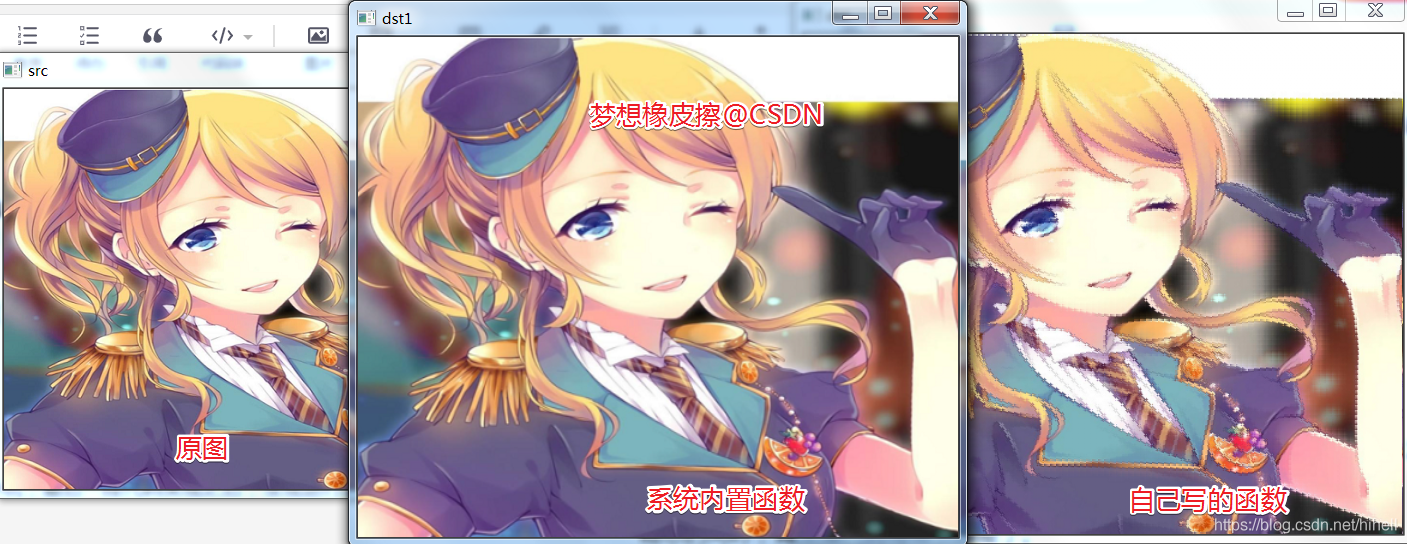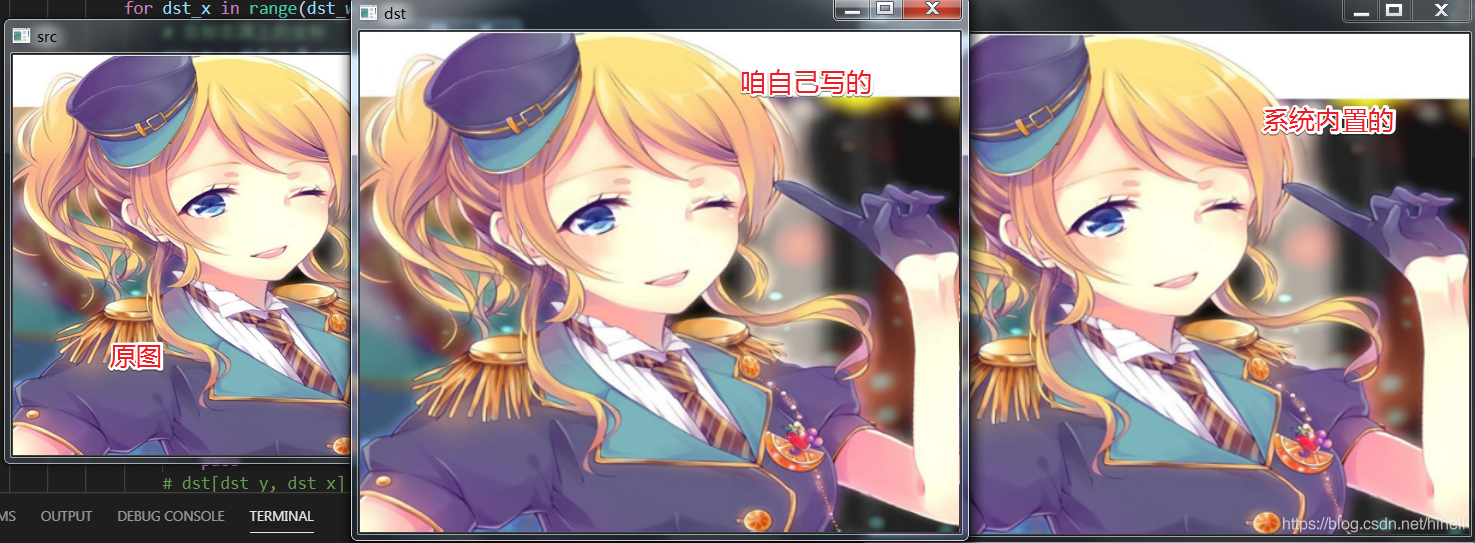# Python OpenCV 图像的 最近邻插值 与 双线性插值算法 优化迭代Python OpenCV 365 天学习计划，与橡皮擦一起进入图像领域吧。本篇博客是这个系列的第 43 篇。

## 基础知识铺垫dst[dst_y, dst_x, n] = (1-u)*(1-v)*src[j, i, n]+u*(1-v) * src[j+1, i, n] + (1-u)*v*src[j, i+1, n] + u*v*src[j+1, i+1, n]

dst[dst_y, dst_x, n] = (1-u)*(1-v)*src[j, i, n]+v*(1-u) * src[j+1, i, n] + (1-v)*u*src[j, i+1, n] + u*v*src[j+1, i+1, n]## 算法优化import cv2 as cvimport numpy as npdef nearest_demo(src, multiple_y, multiple_x):    src_y, src_x, src_c = src.shape    tar_x, tar_y, tar_c = src_x*multiple_x, src_y*multiple_y, src_c    # 生成一个黑色的目标图像    tar_img = np.zeros((tar_y, tar_x, tar_c), dtype=np.uint8)    print(tar_img.shape)    # 渲染像素点的值    # 注意 y 是高度，x 是宽度    for y in range(tar_y-1):        for x in range(tar_x-1):            # 计算新坐标 (x,y) 坐标在源图中是哪个值            src_y = round(y*src_y/tar_y)            src_x = round(x*src_x/tar_x)            tar_img[y, x] = src[src_y, src_x]    return tar_imgsrc = cv.imread("./ttt.png")print(src.shape)cv.imshow("src", src)# dsize = (cols,rows) 中文，(宽度,高度)dst = cv.resize(src, (src.shape*2, src.shape                      * 2), interpolation=cv.INTER_NEAREST)cv.imshow("dst", dst)new_dst = nearest_demo(src, 2, 2)cv.imshow("new_dst", new_dst)cv.waitKey(0)cv.destroyAllWindows()

srcy = round(y*src_y/tar_y)srcx = round(x*src_x/tar_x)# 修改如下srcy = round((y+0.5)*src_y/tar_y-0.5)srcx = round((x+0.5)*src_x/tar_x-0.5)

# 修改前src_y = round(dst_y*src_height/tar_height)src_x = round(dst_x*src_width/tar_width)# 修改后src_y = round(dst_y*src_height/tar_height+1/2*(src_height/tar_height-1))src_x = round(dst_x*src_width/tar_width+1/2*(src_height/tar_height-1))

# 修改后src_y = round((dst_y+0.5)*src_height/tar_height-0.5)src_x = round((dst_x+0.5)*src_width/tar_width-0.5)## 橡皮擦的小节### 梦想橡皮擦

6 年产品经理+教学经验，3 年互联网项目管理经验； 互联网资深爱好者； 沉迷各种技术无法自拔，导致年龄被困在 25 岁； CSDN 爬虫 100 例作者。 个人公众号“梦想橡皮擦”。

## 评论